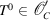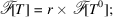International
Tables for
Crystallography
Volume B
Reciprocal space
Edited by U. Shmueli

International Tables for Crystallography (2010). Vol. B, ch. 1.3, pp. 42-43   | 1 | 2 |

## Section 1.3.2.6.4. Fourier transforms of periodic distributions

G. Bricognea

aGlobal Phasing Ltd, Sheraton House, Suites 14–16, Castle Park, Cambridge CB3 0AX, England, and LURE, Bâtiment 209D, Université Paris-Sud, 91405 Orsay, France

#### 1.3.2.6.4. Fourier transforms of periodic distributions

| top | pdf |

The content of this section is perhaps the central result in the relation between Fourier theory and crystallography (Section 1.3.4.2.1.1).

Letwith r defined as in Section 1.3.2.6.2. Then,hence, so that:-periodic distributions are tempered, hence have a Fourier transform. The convolution theorem (Section 1.3.2.5.8) is applicable, giving:and similarly for.

Since, formallysay.

It is readily shown that Q is tempered and periodic, so that, while the periodicity of r implies thatSince the first factors have single isolated zeros atin,(see Section 1.3.2.3.9.4) and hence by periodicity; convoluting withshows that. Thus we have the fundamental result:so thati.e., according to Section 1.3.2.3.9.3,The right-hand side is a weighted lattice distribution, whose nodesare weighted by the sample valuesof the transform of the motifat those nodes. Since, the latter values may be writtenBy the structure theorem for distributions with compact support (Section 1.3.2.3.9.7),is a derivative of finite order of a continuous function; therefore, from Section 1.3.2.4.2.8and Section 1.3.2.5.8,grows at most polynomially as(see also Section 1.3.2.6.10.3about this property). Conversely, letbe a weighted lattice distribution such that the weightsgrow at most polynomially as. Then W is a tempered distribution, whose Fourier cotransformis periodic. If T is now written asfor some, then by the reciprocity theoremAlthough the choice ofis not unique, and need not yield back the same motif as may have been used to build T initially, different choices ofwill lead to the same coefficientsbecause of the periodicity of.

The Fourier transformation thus establishes a duality between periodic distributions and weighted lattice distributions. The pair of relationsare referred to as the Fourier analysis and the Fourier synthesis of T, respectively (there is a discrepancy between this terminology and the crystallographic one, see Section 1.3.4.2.1.1). In other words, any periodic distributionmay be represented by a Fourier series (ii), whose coefficients are calculated by (i). The convergence of (ii) towards T inwill be investigated later (Section 1.3.2.6.10).